### Archive

Posts Tagged ‘Popular Culture’

## Counting and Solving Final Fantasy XIII-2’s Clock Puzzles

February 6th, 2012

Final Fantasy XIII-2 is a role-playing game, released last week in North America, that contains an abundance of mini-games. One of the more interesting mini-games is the “clock puzzle”, which presents the user with N integers arranged in a circle, with each integer being from 1 to$\lfloor N/2 \rfloor$.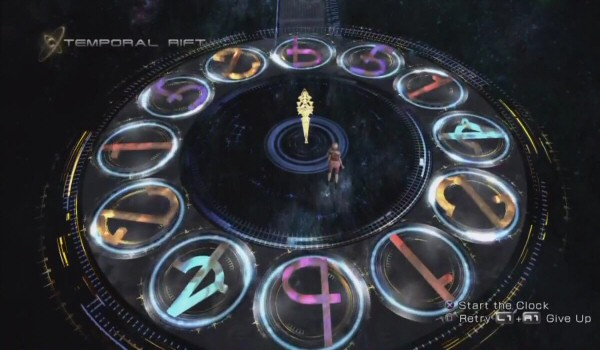A challenging late-game clock puzzle with N = 12

The way the game works is as follows:

1. The user may start by picking any of the N positions on the circle. Call the number in this position M.
2. You now have the option of picking either the number M positions clockwise from your last choice, or M positions counter-clockwise from your last choice. Update the value of M to be the number in the new position that you chose.
3. Repeat step 2 until you have performed it N-1 times.

You win the game if you choose each of the N positions exactly once, and you lose the game otherwise (if you are forced to choose the same position twice, or equivalently if there is a position that you have not chosen after performing step 2 a total of N-1 times). During the game, N ranges from 5 to 13, though N could theoretically be as large as we like.

### Example

To demonstrate the rules in action, consider the following simple example with N = 6 (I have labelled the six positions 05 in blue for easy reference):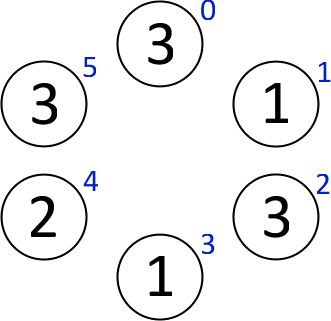If we start by choosing the 1 in position 1, then we have the option of choosing the 3 in either position 0 or 2. Let’s choose the 3 in position 0. Three moves either clockwise or counter-clockwise from here both give the 1 in position 3, so that is our only possible next choice. We continue on in this way, going through the N = 6 positions in the order 103425, as in the following image: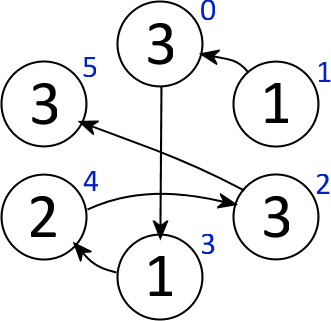We have now selected each position exactly once, so we are done – we solved the puzzle! In fact, this is the unique solution for the given puzzle.

### Counting Clock Puzzles

Let’s work on determining how many different clock puzzles there are of a given size. As mentioned earlier, a clock puzzle with N positions has an integer in the interval$[1, \lfloor N/2 \rfloor]$ in each of the  positions. There are thus$\lfloor N/2 \rfloor^N$ distinct clock puzzles with N positions, which grows very quickly with N – its values for N = 1, 2, 3, … are given by the sequence 0, 1, 1, 16, 32, 729, 2187, 65536, 262144, … (A206344 in the OEIS).

However, this rather crude count of the number of clock puzzles ignores the fact that some clock puzzles have no solution. To illustrate this fact, we present the following simple proposition:

Proposition. There are unsolvable clock puzzles with N positions if and only if N = 4 or N ≥ 6.

To prove this proposition, first note that the clock puzzles for N = 2 or N = 3 are trivially solvable, since each number in the puzzle is forced to be$\lfloor N/2 \rfloor = 1$. The 32 clock puzzles in the N = 5 case can all easily be shown to be solvable via computer brute force (does anyone have a simple or elegant argument for this case?).

In the N = 4 case, exactly 3 of the 16 clock puzzles are unsolvable: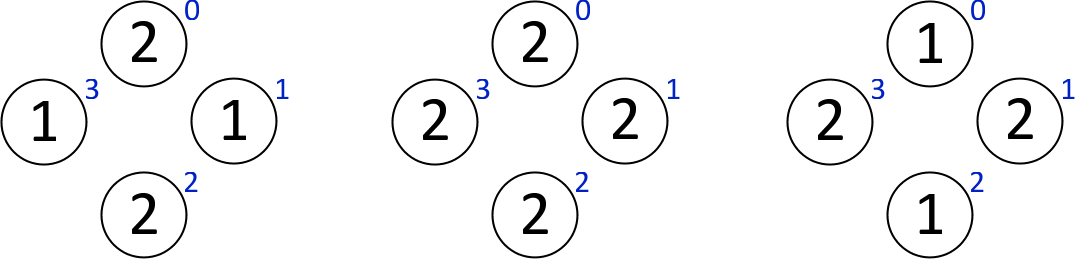To complete the proof, it suffices to demonstrate an unsolvable clock puzzle for each N ≥ 6. To this end, we begin by considering the following clock puzzle in the N = 6 case: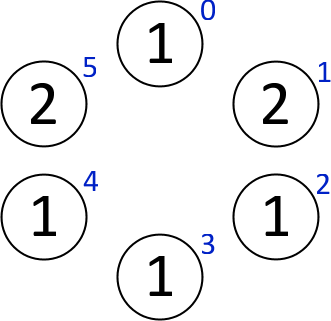The above puzzle is unsolvable because the only way to reach position 0 is to select it first, but from there only one of positions 2 or 4 can be reached – not both. This example generalizes in a straightforward manner to any N ≥ 6 simply by adding more 1’s to the bottom: it will still be necessary to choose position 0 first, and then it is impossible to reach both position 2 and position N-2 from there.

There doesn’t seem to be an elegant way to count the number of solvable clock puzzles with N positions (which is most likely related to the apparent difficulty of solving these puzzles, which will be discussed in the next section), so let’s count the number of solvable clock puzzles via brute force. Simply constructing each of the$\lfloor N/2 \rfloor^N$ clock puzzles and determining which of them are solvable (via the MATLAB script linked at the end of this post) shows that the number of solvable clock puzzles for N = 1, 2, 3, … is given by the sequence 0, 1, 1, 13, 32, 507, 1998, 33136, 193995, … (A206345 in the OEIS).

This count of puzzles is perhaps still unsatisfying, though, since it counts puzzles that are simply mirror images or rotations of each other multiple times. Again, there doesn’t seem to be an elegant counting argument for enumerating the solvable clock puzzles up to rotation and reflection, so we compute this sequence by brute force: 0, 1, 1, 4, 8, 72, 236, 3665, 19037, … (A206346 in the OEIS).

### Solving Clock Puzzles

Clock puzzles are one of the most challenging parts of Final Fantasy XIII-2, and with good reason: they are a well-studied graph theory problem in disguise. We can consider each clock puzzle with N positions as a directed graph with N vertices. If position N contains the number M, then there is a directed edge going from vertex N to the vertices M positions clockwise and counter-clockwise from it. In other words, we consider a clock puzzle as a directed graph on N vertices, where the directed edges describe the valid moves around the circle.The directed graph corresponding to the earlier (solvable) N = 6 example

The problem of solving a clock puzzle is then exactly the problem of finding a directed Hamiltonian path on the associated graph. Because finding a directed Hamiltonian path in general is NP-hard, this seems to suggest that solving clock puzzles might be as well. There of course is the problem that the directed graphs relevant to this problem have very special structure – in particular, every vertex has outdegree ≤ 2, and the graph has a symmetry property that results from clockwise/counter-clockwise movement allowed in the clock puzzles.

The main result of  shows that the fact that the outdegree of each vertex is no larger than 2 is no real help: finding directed Hamiltonian paths is still NP-hard given such a promise. However, the symmetry condition seems more difficult to characterize in graph theoretic terms, and could potentially be exploited to produce a fast algorithm for solving these puzzles.

Regardless of the problem’s computational complexity, the puzzles found in the game are quite small (N ≤ 13), so they can be easily solved by brute force. Attached is a MATLAB script (solve_clock.m) that can be used to solve clock puzzles. The first input argument is a vector containing the numeric values in each of the positions, starting from the top and reading clockwise. By default, only one solution is computed. To compute all solutions, set the second (optional) input argument to 1.

The output of the script is either a vector of positions (labelled 0 through N-1, with 0 referring to the top position, 1 referring to one position clockwise from there, and so on) describing an order in which you can visit the positions to solve the puzzle, or 0 if there is no solution.

For example, the script can be used to find our solution to the N = 6 example provided earlier:

```>> solve_clock([3,1,3,1,2,3])

ans =
1 0 3 4 2 5```

Similarly, the script can be used to find all four solutions [Update, October 1, 2013: Whoops, there are six solutions! See the comments.] to the puzzle in the screenshot at the very top of this post:

```>> solve_clock([6,5,1,4,2,1,6,4,2,1,5,2], 1)

ans =
3 7 11 9 10 5 4 2 1 8 6 0
7 3 11 9 10 5 4 2 1 8 6 0
9 10 5 4 2 3 7 11 1 8 6 0
9 8 10 5 4 2 3 7 11 1 6 0```

References

1. J. Plesnik. The NP-completeness of the Hamiltonian cycle problem in planar digraphs with degree bound two. Inform. Process. Lett., 8:199–201, 1979.

## The Maximum Score in the Game “Entanglement” is 9080

January 21st, 2011

Entanglement is a browser-based game that has gained a fair bit of popularity lately due to its recent inclusion in Google’s Chrome Web Store and Chrome 9. The way the game works is probably best understood by actually playing it, but here is my brief attempt:

• You are given a hexagonal tile with six paths printed on it, with two path ends touching each side of the hexagon. One such tile is as follows:• You may rotate, but not move the hexagon that has been provided to you.
• Once you have selected an orientation of the hexagon, a path is traced along that hexagon, and you are provided a new hexagon that you may rotate at the end of your current path.
• The goal of the game is to create the longest path possible without running into either the centre hexagon or the outer edge of the game board.

To make things a bit more interesting, the game was updated in November 2010 to include a new scoring system that gives you 1 + 2 + 3 + … + n (the nth triangular number) points on a turn if you extend the length of your path by n on that turn. This encourages clever moves that significantly extend the length of the path all at once. The question that I am going to answer today is what the maximum score in Entanglement is under this scoring system (inspired by this reddit thread).

### On a Standard-Size Game Board

The standard Entanglement game board is made up of a hexagonal ring of 6 hexagons, surrounded by a hexagonal ring of 12 hexagons, surrounded by a hexagonal ring of 18 hexagons, for a total of 36 hexagons. In order to maximize our score, we want to maximize how much we increase the length of our path on our final move. Thus, we want to just extend our path by a length of one on each of our first 35 moves, and then score big on the 36th move.

Well, each hexagon that we lay has six paths on it, for a total of 6*36 = 216 paths on the board. 35 of those paths will be used up by our first 35 moves. It is not possible to use all of the remaining 181 paths, however, because many of them lead into the edge of the game board or the central hexagon, and connecting to such a path immediately ends the game. Because there are 12 path ends that touch the central hexagon and 84 path ends that touch the outer border, there must be at least (12+84)/2 – 1 = 47 unused paths on the game board (we divided by 2 because each unused path takes up two path ends and we subtracted 1 because one of the paths will be used by us).

Thus we can add a length of at most 181 – 47 = 134 to our path on the 36th and final move of the game, giving a total score of at most 35 (from the first 35 moves of the game) + 1 + 2 + 3 + … + 134 = 35 + 9045 = 9080. Not only is this an upper bound of the possible scores, but it is actually attainable, as demonstrated by the following optimal game board:Paths in red are unused, the green line depicts the portion of the path laid by the first 35 moves of the game, and the blue line depicts the portion of the path (of length 134) gained on the 36th move. One fun property of the above game board is that it is actually completely “unentangled” – no paths cross over any other paths.

### On a Larger or Smaller Game Board

Other than being a good size for playability purposes, there is no reason why we couldn’t play Entanglement on a game board of larger or smaller radius (by radius I mean the number of rings of hexagons around the central hexagon – the standard game board has a radius of 3). We will compute the maximum score simply by mimicking our previous analysis for the standard game board. If the board has radius n, then there are 6 + 12 + 18 + … + 6n = 3n(n+1) hexagons, each of which contains 6 paths. Thus there are 18n(n+1) lengths of path, 3n(n+1)-1 of which are used in the first 3n(n+1)-1 moves of the game, and we want to add as many as possible of the remaining 15n(n+1)+1 lengths of path in the final move of the game. There are 12 path ends that touch the central hexagon and 12 + 24n path ends that touch the outer edge of the game board. Thus there are at least (12 + 12 + 24n)/2 – 1 = 11 + 12n unused paths on the game board.

Tallying the numbers up, we see that on the final move, we can add at most 15n(n+1)+1 – (11 + 12n) = 15n2 + 3n – 10 lengths of path. If T(n) = n(n+1)/2 is the nth triangular number, then we see that it’s not possible to obtain more than 3n(n+1)-1 + T(15n2 + 3n – 10) = (225/2)n4 + 45n3 – 135n2 – (51/2)n + 44 points. In fact, this score is obtainable via the exact same construction as the optimal board in the n = 3 case – just extend the (counter)clockwise rotation of the path in the obvious way. Thus, the maximum score for a game of Entanglement on a board of radius n for n = 1, 2, 3, … is given by the sequence 41, 1613, 9080, 29462, 72479, … (A180667 in the OEIS).

## Statistical Analysis of Password Strength via Gawker’s Leaked Database

December 15th, 2010

This past weekend, Gawker Media was hacked and its user account database was leaked online. The database contained about 1.3 million rows of information containing usernames, e-mail addresses, and passwords (encrypted via DES). This security breach is unfortunate for people whose information is contained within that database, but the silver lining is that it provides a rare opportunity for statistics nerds like me to analyze some otherwise completely unobtainable data.

Because the passwords were encrypted using such an out-of-date scheme (tsk, tsk, Gawker), about 200,000 of the passwords contained in the database have been decrypted. Of course, the passwords that were cracked were relatively weak. For example, all 2641 accounts that used some trivial modification of “password” or “querty” as their password were of course decrypted. In this post I will look at trends in which users’ passwords were cracked to gain insight into which users do and do not create strong passwords.

It should of course be made clear that, because this data comes from a single database, the results that follow may not be representative of the population as a whole, but rather may be skewed by the fact that people with Gawker accounts are generally more “techy” than the average internet user.

### Preliminaries: Cleaning Up the Database

The database of course had to be significantly cleaned before it could be of too much use statistically, so some of the numbers here may differ slightly from the raw numbers you see from news outlets or if you download the raw database yourself. The numbers here are the result of removing any incomplete rows from the database (i.e., rows missing a password, e-mail address, or both) and removing any accounts that were clearly created by SPAMbots (I’m only interested in the password strength of real users).

Also, I will only look at accounts that contain an e-mail address with a domain that was registered in the database at least 50 times. This restriction is in place partly because it is extremely difficult to compute any sort of meaningful statistics on something with a sample size that is much smaller than 50, and it is partly due to the fact that Gawker doesn’t require verified e-mail addresses (so 46993 of the 52593 domain names listed in the database were used by exactly one person, many of which are clearly fake and/or for SPAM).

After making the aforementioned “fixes” to the database, there are 412670 accounts, 157794 (38.2%) of which had their password decrypted.

### Password Strength by Domain Name

The following table displays the 10 most frequently-occurring domain names used for e-mail addresses in the database along with how many users of the domain had their password cracked.

Domain Total Accounts Decrypted Passwords Decryption %
gmail.com 158031 50530 32.0%
yahoo.com 94147 40964 43.5%
hotmail.com 66752 27332 40.9%
aol.com 17534 8151 46.5%
comcast.net 7222 2801 38.8%
msn.com 5544 2250 40.6%
mac.com 4951 1750 35.3%
sbcglobal.net 3896 1667 42.8%
hotmail.co.uk 3204 1476 46.1%
verizon.net 2211 860 38.9%

The following table shows the z-values associated with the statistical test that the two given domains have the same proportion of users with strong passwords. Differences that are statistically significant at the α = 0.01 level are in bold. Click on a z-value to see a normal distribution showing the associated p-value. Notice in particular that gmail.com users have stronger passwords than users of any of the other top-10 domain names, while aol.com and hotmail.co.uk users have the weakest passwords.

Yahoo Hotmail AOL Comcast MSN Mac SBC HotmailUK Verizon
GMail 58.28 40.84 38.65 12.10 13.48 5.00 14.27 16.89 6.92
Yahoo -10.26 7.29 -7.81 -4.27 -11.31 -0.89 2.87 -4.33
Hotmail 13.23 -3.55 -0.53 -7.74 2.27 5.75 -1.93
AOL -11.09 -7.70 -13.94 -4.19 -0.44 -6.75
Comcast 2.06 -3.85 4.11 6.98 0.09
MSN -5.52 2.14 5.00 -1.37
Mac 7.14 9.67 2.88
SBC 2.77 -2.97
HotmailUK -5.24

### Educational Institutions

Not surprisingly, users who entered an e-mail address from an educational institution typically had stronger passwords than the general population. Of the 2092 users who provided a college or university-based e-mail address, only 697 (33.3%) were decrypted. This proportion is significantly lower than the corresponding proportion for the general population (z = 4.64, p < 0.001).

However, two universities stood out as having particularly weak passwords: of the 56 users who used a University of Texas e-mail address, 27 (48.2%) had their password decrypted, and similarly 101 (45.1%) of 224 New York University passwords were decrypted.

### ISP-Provided E-Mail Users

Users who used an e-mail address provided to them by their ISP (such as something@comcast.net) typically had weaker passwords than the general population, a fact that can perhaps be explained by the fact that tech-unsavvy folks are less likely to go out and get a new e-mail address for themselves at a place like GMail. Of the 31667 users who provided an ISP-based e-mail address, 13053 (41.2%) of them had their password decrypted. This proportion is significantly higher than the corresponding proportion for the general population (z = -11.36, p < 0.001).

Also unsurprisingly, users who entered an obvious typo in their e-mail address were much more likely to have a weak password than people who entered their e-mail address correctly (by “obvious typo” I basically mean an e-mail address containing a typo of a common domain name, such as “fred@yahoo,com” or “fred@hotmail”). Of the 530 users with a typo in their e-mail address, 280 (52.8%) had passwords that were decrypted. This proportion is significantly higher than the average (z = -6.87, p < 0.001).

The following table shows the strength of user passwords based on the country associated with their e-mail address. Of course some e-mail addresses provide no information about the user’s country, so domains that serve a largely international market (such as gmail.com, mac.com and aim.com) are excluded from this analysis.

Country Total Accounts Decrypted Passwords Decryption %
India 3129 1448 46.3%
United Kingdom 6874 3057 44.5%
China 1411 600 42.5%
United States 30891 12507 40.5%
Germany 1378 484 35.1%
Russia 2223 533 24.0%

So Russia and Germany are the big winners when it comes to password strength, while India and the United Kingdom seem to have the weakest passwords. The following table shows the z-values associated with the statistical test that the two given countries have the same proportion of users with strong passwords. Differences that are statistically significant at the α = 0.01 level are in bold. Click on a z-value to see a normal distribution showing the associated p-value.

UK China Canada US Germany Russia
India -1.67 -2.32 -4.03 -6.26 -6.94 -16.62
UK -1.31 -3.06 -6.05 -6.37 -17.16
China -0.88 -1.49 -3.97 -11.72